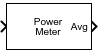# Power Meter

Measure power of voltage signal

• Library:
• DSP System Toolbox / Statistics

•## Description

The Power Meter block computes the power measurements of a voltage signal. The power measurements include average power, peak power, and peak-to-average power ratio. The block uses the sliding window method to compute these measurements. For more details, see Algorithms.

## Ports

### Input

expand all

Specify the input signal in volts as a vector or a matrix. If `x` is a matrix, each column is treated as an independent channel. The power measurement is computed along each channel using the Sliding Window Method.

Data Types: `single` | `double`
Complex Number Support: Yes

### Output

expand all

Average power of the voltage signal, returned as a vector or a matrix, and measured in the units determined by the Power units parameter. For details on how the block computes the average power, see Average Power.

#### Dependencies

To enable this port, set Measurement to `Average power` or `All`.

Data Types: `single` | `double`

Peak power of the voltage signal, returned as a vector or a matrix, and measured in the units determined by the Power units parameter. For details on how the block computes the peak power, see Peak Power.

#### Dependencies

To enable this port, set Measurement to ```Peak power``` or `All`.

Data Types: `single` | `double`

Peak-to-average power ratio of the voltage signal, returned as a vector or a matrix. For details on how the block computes the peak-to-average power ratio, see Peak-to-Average Power Ratio.

#### Dependencies

To enable this port, set Measurement to `Peak-to-average power ratio` or `All`.

Data Types: `single` | `double`

## Parameters

expand all

Specify the desired power measurement as one of the following:

• `Average power` (default)

• `Peak power`

• `Peak-to-average power ratio`

• `All`

For details on how the block computes these measurements, see Algorithms.

Specify the window length over which the measurement is computed as a positive integer.

Specify the reference load that the power meter uses to compute power values as a real, positive scalar in ovohms.

Tunable: Yes

Specify the units of the measured power values as one of the following:

• `dBm`

• `dBW`

• `Watts`

• `Code generation`: Simulate model using generated C code. The first time you run a simulation, Simulink® generates C code for the block. The C code is reused for subsequent simulations, as long as the model does not change. This option requires additional startup time but provides faster simulation speed than `Interpreted execution`.

• `Interpreted execution`: Simulate model using the MATLAB®  interpreter. This option shortens startup time but has slower simulation speed than `Code generation`.

## Block Characteristics

 Data Types `double` | `single` Direct Feedthrough `no` Multidimensional Signals `no` Variable-Size Signals `yes` Zero-Crossing Detection `no`

expand all

## Extended Capabilities

### Blocks

Introduced in R2021a

## SupportGet trial now